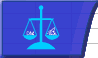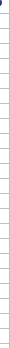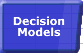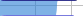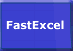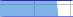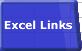# Sum with Multiple ConditionsImportance of Speed Faster Calculations Microsoft's Advice Bottlenecks/Size Lookups Dynamic Ranges Totalling Generating Workbooks Multi-Level Calculations Array Formulae SUMIF Example Worksheet Links## SUM with Multiple Conditions

The SUMIF function only allows a single condition. Often you need to do SUM calculations that are dependent on multiple conditions. These calculations can be very sensitive to the method of calculation used.

 This example shows how to reduce the calculation time for this problem from several hours to a tenth of a second. The example below shows successive optimisation of a calculation using SUM with multiple conditions. See also the FastExcel Sample Problem Download. The different approaches used have calculation times ranging between 3 hours to 0.14 seconds. Each approach gives identical results. A number of sites act as origins and destinations in a network. There are 10000 origin-destination pairs called routes. Each route has a shipment volume for each of 5 years. Each origin and destination is graded as A or B. Each origin site always has additional destinations that start with OTH. We need to calculate a coverage factor for each route for each of the 5 years as follows: Factor for a route=(sum of volumes for all the routes starting at this routes origin and with both origin and destination=A)/(total volume for this routes origin – volumes from this routes origin to destinations starting with OTH) The Conditional Sum Wizard is easy to use but produces slow calculations. :Over 3 hours. The easiest approach is to use Excel’s built-in Conditional Sum Wizard (you can install this using Tools->Add-Ins). The Conditional Sum Wizard generates an array formula using nested ifs, for example: {SUM(IF(\$A\$4:\$A\$10003=\$A4,IF(L\$4:L\$10003=”AA”, D\$4:D\$10003,0),0))} This calculation for the 10000 routes takes 744 seconds on my 500 MHZ laptop. To solve the complete problem would need another 15 similar columns of formulae, and the calculation time would be over 3 hours.
 Using an Array formula is faster but still too slow. An alternative approach is to use multiplicative AND’s in the array formula: {SUM((\$A\$4:\$A\$10003=\$A4)*(L\$4:L\$10003=”AA”)* (D\$4:D\$10003))} This calculation is slightly faster at 723 seconds for a single column against 744, but still far too slow. Using SUMPRODUCT is faster than an array formula, but not enough. Or you can use SUMPRODUCT, which does not need to be array-enetered: SUMPRODUCT(--(\$A\$4:\$A\$10003=\$A4),--(L\$4:L\$10003="AA"),D\$4:D\$10003) SUMPRODUCT is faster than the array formula, 711 seconds compared to the 723, but its still too slow. Calculate once, store the results and then lookup the results many times. 89 Seconds. With this calculation all routes with the same origin have the same answer: so we can reduce the calculation time significantly by only doing it once for each of the 100 unique origins, and then looking up the answer for each of the 10000 routes. The following formulae do the calculation for one year at origin level in 17.8 seconds, or 5 years in 89 seconds. This is much better but still too slow. {SUM((RouteCalc!\$A\$4:\$A\$10003=\$A5)* (RouteCalc!\$L\$4:\$L\$10003="AA")* (RouteCalc!D\$4:D\$10003))} SUM((RouteCalc!\$A\$4:\$A\$10003=\$A5)* (LEFT(RouteCalc!\$B\$4:\$B\$10003,3)="OTH")* (RouteCalc!D\$4:D\$10003)) SUMIF(RouteCalc!\$A\$4:\$A\$10003,A5,RouteCalc!D\$4:D\$10003) E5/(G5-F5) Dynamically calculate subset blocks to be used in the calculation. 0 .7 seconds These formulae do not exploit the fact that the routes are sorted by destination within origin, so that the block of rows we need to do the SUM over for each origin is contiguous and much smaller than 10000 rows. However we need to be aware that the origin and destination Sites could change. We can calculate the range to use in the formulae using three extra columns: The number of routes for this origin (numrows) is: COUNTIF(RouteCalc!\$A\$4:\$A\$10003,A5) The row number of the last route for this origin is the start row for this origin plus the number of routes. The row number if the first route for the next origin (startrow) is the previous origin’s last route row number plus 1. The coverage formulae then do not need array formulae and can use SUMIF and OFFSET as follows: SUMIF(OFFSET(RouteCalc!\$K\$1,startrow,1,numrows,1), "AA",OFFSET(RouteCalc!\$C\$1,startrow,1,numrows,1)) SUMIF(OFFSET(RouteCalc!\$B\$1,startrow,0,numrows,1), "oth*",OFFSET(RouteCalc!\$C\$1,startrow,1,numrows,1)) SUM(OFFSET(RouteCalc!\$C\$1,startrow,1,numrows,1)) E5/(G5-F5) Calculation time for all 5 years is now 0.673 seconds. Dynamically calculate a small subset block for the COUNTIF. 0 .14 seconds Analysis of these calculations shows that over 80% of the time is being used by the COUNTIF formulae. We can reduce this using the fact that there will never be more than 300 origin Sites in this model, (it would be better still to count the number of unique Sites). COUNTIF(OFFSET(RouteCalc!\$A\$1,PreviousOriginLastRow,0,300),A7) This reduces the calculation time to 0.14 seconds.

So the calculation time has been reduced from 3 hours to 0.14 seconds, which is an improvement factor of over 77000!© 2001-2020 Decision Models Legal Disclaimer Privacy Statement